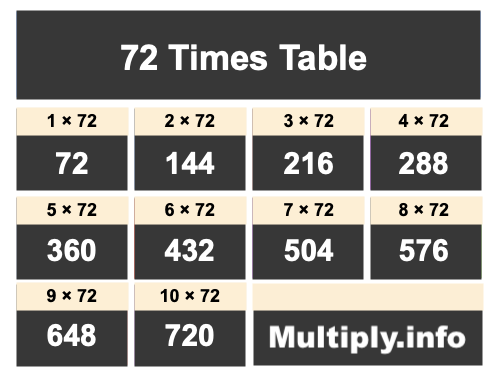72 Times TableThe picture above shows the first ten numbers in the 72 Times Table. It is created my multiplying 72 by one, then by two, then by three, and so on all the way up to ten.

Students and others use our 72 Times Table for many things, such as to find patterns or for memorizing products of numbers multiplied by 72.

In addition to the image of the 72 Times Table above, we have also written the 72 Times Table below showing 72 multiplied by every number up to one hundred. The list is in ascending order.

We created the 72 Times Table below to make it easy to read and print. We hope you will find our 72 Times Table helpful.

72 x  0 = 0
72 x  1 = 72
72 x  2 = 144
72 x  3 = 216
72 x  4 = 288
72 x  5 = 360
72 x  6 = 432
72 x  7 = 504
72 x  8 = 576
72 x  9 = 648
72 x 10 = 720
72 x 11 = 792
72 x 12 = 864
72 x 13 = 936
72 x 14 = 1008
72 x 15 = 1080
72 x 16 = 1152
72 x 17 = 1224
72 x 18 = 1296
72 x 19 = 1368
72 x 20 = 1440
72 x 21 = 1512
72 x 22 = 1584
72 x 23 = 1656
72 x 24 = 1728
72 x 25 = 1800
72 x 26 = 1872
72 x 27 = 1944
72 x 28 = 2016
72 x 29 = 2088
72 x 30 = 2160
72 x 31 = 2232
72 x 32 = 2304
72 x 33 = 2376
72 x 34 = 2448
72 x 35 = 2520
72 x 36 = 2592
72 x 37 = 2664
72 x 38 = 2736
72 x 39 = 2808
72 x 40 = 2880
72 x 41 = 2952
72 x 42 = 3024
72 x 43 = 3096
72 x 44 = 3168
72 x 45 = 3240
72 x 46 = 3312
72 x 47 = 3384
72 x 48 = 3456
72 x 49 = 3528
72 x 50 = 3600
72 x 51 = 3672
72 x 52 = 3744
72 x 53 = 3816
72 x 54 = 3888
72 x 55 = 3960
72 x 56 = 4032
72 x 57 = 4104
72 x 58 = 4176
72 x 59 = 4248
72 x 60 = 4320
72 x 61 = 4392
72 x 62 = 4464
72 x 63 = 4536
72 x 64 = 4608
72 x 65 = 4680
72 x 66 = 4752
72 x 67 = 4824
72 x 68 = 4896
72 x 69 = 4968
72 x 70 = 5040
72 x 71 = 5112
72 x 72 = 5184
72 x 73 = 5256
72 x 74 = 5328
72 x 75 = 5400
72 x 76 = 5472
72 x 77 = 5544
72 x 78 = 5616
72 x 79 = 5688
72 x 80 = 5760
72 x 81 = 5832
72 x 82 = 5904
72 x 83 = 5976
72 x 84 = 6048
72 x 85 = 6120
72 x 86 = 6192
72 x 87 = 6264
72 x 88 = 6336
72 x 89 = 6408
72 x 90 = 6480
72 x 91 = 6552
72 x 92 = 6624
72 x 93 = 6696
72 x 94 = 6768
72 x 95 = 6840
72 x 96 = 6912
72 x 97 = 6984
72 x 98 = 7056
72 x 99 = 7128
72 x 100 = 7200

Times Table Charts
Enter another number below to see the Times Table for that number.

73 Times Table
Did you find useful patterns in our 72 Times Table that you can use for the future? Check out the next Times Table on our list.